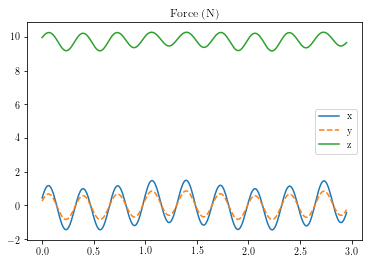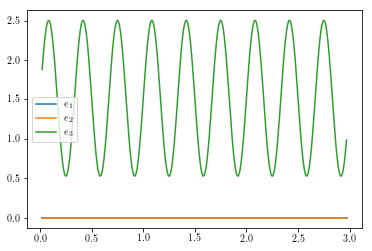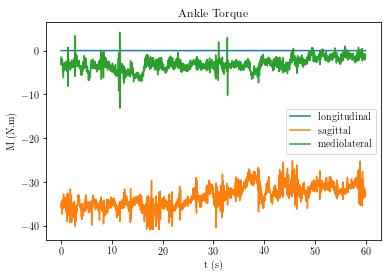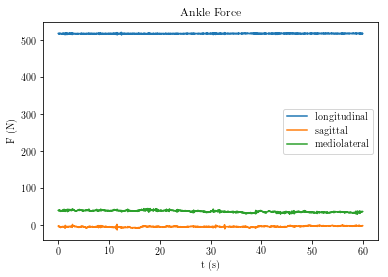# Three-dimensional rigid body kinetics¶

Renato Naville Watanabe

## The Newton-Euler laws¶

The Newton-Euler laws remain valid in the three-dimensional motion of a rigid body (for revision on Newton-Euler laws, see this notebook about these laws).

\begin{equation} \vec{\bf{F}} = m\vec{\bf{a_{cm}}} \end{equation}\begin{equation} \vec{\bf{M_O}} = \frac{d\vec{\bf{H_O}}}{dt} \end{equation}

## Resultant force and moments¶

The resultant force and the resultant moment around a point O are computed in the same way of the two-dimensional case.

\begin{equation} \vec{\bf{F}} = \sum\limits_{i=1}^n \vec{\bf{F_n}} \end{equation}\begin{equation} \vec{\bf{M_O}} = \sum\limits_{i=1}^n \vec{\bf{r_{i/O}}} \times \vec{\bf{F_i}} \end{equation}

## Deduction of the angular momentum in three-dimensional movement¶

The major difference that appears when we are dealing with three-dimensional motions is to compute the derivative of the angular momentum. For the sake of simplicity, the analysis will only consider the point O as the center of mass of the body.

We begin computing the angular momentum of a rigid body around its center of mass.

\begin{equation} \vec{\boldsymbol{H_{cm}}} = \int_B \vec{\boldsymbol{r_{/cm}}} \times \vec{\boldsymbol{v}}\,dm = \int \vec{\boldsymbol{r_{/cm}}} \times (\vec{\boldsymbol{\omega}}\times\vec{\boldsymbol{r_{/cm}}})\,dm \end{equation}

where $\vec{\boldsymbol{r_{/cm}}}$ is the vector from the point O to the position of the infinitesimal mass considered in the integral. For simplicity of the notation, we will use $\vec{\boldsymbol{r}} = \vec{\boldsymbol{r_{/cm}}}$.

So, the angular momentum is:

$$\vec{\boldsymbol{H_{cm}}} = \int \vec{\boldsymbol{r}} \times (\vec{\boldsymbol{\omega}}\times\vec{\boldsymbol{r}})\,dm = \int (r_x\hat{\boldsymbol{i}} + r_y\hat{\boldsymbol{j}}+r_z\hat{\boldsymbol{k}}) \times \left[(\omega_x\hat{\boldsymbol{i}} + \omega_y\hat{\boldsymbol{j}}+\omega_z\hat{\boldsymbol{k}})\times(r_x\hat{\boldsymbol{i}} + r_y\hat{\boldsymbol{j}}+r_z\hat{\boldsymbol{k}})\right]\,dm$$
$$\vec{\boldsymbol{H_{cm}}}= \int (r_x\hat{\boldsymbol{i}} + r_y\hat{\boldsymbol{j}}+r_z\hat{\boldsymbol{k}}) \times \left[(\omega_x r_y\hat{\boldsymbol{k}} - \omega_xr_z\hat{\boldsymbol{j}} - \omega_yr_x\hat{\boldsymbol{k}} + \omega_yr_z\hat{\boldsymbol{i}}+ \omega_zr_x\hat{\boldsymbol{j}}- \omega_zr_y\hat{\boldsymbol{i}}\right]\,dm$$
$$\vec{\boldsymbol{H_{cm}}}= \int-\omega_x r_xr_y\hat{\boldsymbol{j}} -\omega_x r_xr_z\hat{\boldsymbol{k}} +\omega_y r_x^2\hat{\boldsymbol{j}} + \omega_zr_x^2\hat{\boldsymbol{k}}+ \omega_xr_y^2\hat{\boldsymbol{i}} -\omega_yr_xr_y\hat{\boldsymbol{i}}-\omega_yr_yr_z\hat{\boldsymbol{k}}+\omega_zr_y^2\hat{\boldsymbol{k}}+\omega_x r_z^2\hat{\boldsymbol{i}} +\omega_y r_z^2\hat{\boldsymbol{j}} -\omega_z r_xr_z\hat{\boldsymbol{i}} - \omega_zr_yr_z\hat{\boldsymbol{j}}\,dm$$
$$\vec{\boldsymbol{H_{cm}}}=\left(\int \omega_xr_y^2 -\omega_yr_xr_y+\omega_x r_z^2-\omega_z r_xr_z\,dm\right)\;\hat{\boldsymbol{i}}+\left(\int-\omega_x r_xr_y +\omega_y r_x^2 +\omega_y r_z^2 - \omega_zr_yr_z\,dm\right)\hat{\boldsymbol{j}}+\left(\int-\omega_x r_xr_z + \omega_zr_x^2-\omega_yr_yr_z+\omega_zr_y^2 \,dm\right)\hat{\boldsymbol{k}}$$
$$\vec{\boldsymbol{H_{cm}}}=\left(\int \omega_x(r_y^2+r_z^2)\,dm+ \int-\omega_yr_xr_y\,dm + \int-\omega_z r_xr_z\,dm\right)\;\hat{\boldsymbol{i}} + \left(\int-\omega_x r_xr_y\,dm +\int\omega_y (r_x^2 +r_z^2)\,dm + \int- \omega_zr_yr_z\,dm\right)\hat{\boldsymbol{j}} + \left(\int-\omega_x r_xr_z\,dm + \int-\omega_yr_yr_z\,dm + \int \omega_z(r_x^2+r_y^2) \,dm\right)\hat{\boldsymbol{k}}$$
$$\vec{\boldsymbol{H_{cm}}}=\bigg(\omega_x\int (r_y^2+r_z^2)\,dm+ \omega_y\int-r_xr_y\,dm + \omega_z\int- r_xr_z\,dm\bigg)\;\hat{\boldsymbol{i}}+\bigg(\omega_x\int- r_xr_y\,dm +\omega_y\int (r_x^2 +r_z^2)\,dm + \omega_z\int-r_yr_z\,dm\bigg)\hat{\boldsymbol{j}} + \bigg(\omega_x\int- r_xr_z\,dm + \omega_y\int-r_yr_z\,dm + \omega_z\int (r_x^2+r_y^2) \,dm\bigg)\hat{\boldsymbol{k}}$$
$$\vec{\boldsymbol{H_{cm}}}=\left(\omega_xI_{xx}^{cm}+ \omega_yI_{xy}^{cm} + \omega_zI_{xz}^{cm}\right)\;\hat{\boldsymbol{i}} + \left(\omega_xI_{xy}^{cm} +\omega_yI_{yy}^{cm} + \omega_zI_{yz}^{cm}\right)\hat{\boldsymbol{j}}+\left(\omega_xI_{yz}^{cm} + \omega_yI_{yz}^{cm} + \omega_zI_{zz}^{cm}\right)\hat{\boldsymbol{k}}$$

## Angular momentum in three-dimensional movement¶

\begin{align} \begin{split} \vec{\boldsymbol{H_{cm}}}=\left[\begin{array}{ccc}I_{xx}^{cm}&I_{xy}^{cm}&I_{xz}^{cm}\\ I_{xy}^{cm}&I_{yy}^{cm}&I_{yz}^{cm}\\ I_{xz}^{cm}&I_{yz}^{cm}&I_{zz}^{cm}\end{array}\right]\cdot \left[\begin{array}{c}\omega_x\\\omega_y\\\omega_z \end{array}\right] = I\vec{\boldsymbol{\omega}} \end{split} \label{eq:angmom} \end{align}

where this matrix is the Matrix of Inertia (or more rigorously, Tensor of Inertia) as defined previously in this notebook about moment of inertia.

## The matrix of inertia is different depending on the body orientation¶

So, to compute the angular momentum of a body we have to multiply the matrix of inertia $I$ of the body by its angular velocity $\vec{\boldsymbol{\omega}}$. The problem on this approach is that the moments and products of inertia depends on the orientation of the body relative to the frame of reference. As they depend on the the distances of each point of the body to the axes, if the body is rotating, the matrix of inertia $I$ will be different at each instant.

## The solution is to attach a frame reference to the body¶

The solution to this problem is to attach a frame of reference to the body. We will denote this frame of reference as $\hat{\boldsymbol{e_1}}$, $\hat{\boldsymbol{e_2}}$ and $\hat{\boldsymbol{e_3}}$, with origin in the center of mass of the body. As can be noted from the figure below, the frame of reference moves along the body. Now the matrix of inertia $I$ will be constant relative to this new basis $\hat{\boldsymbol{e_1}}$, $\hat{\boldsymbol{e_2}}$ and $\hat{\boldsymbol{e_3}}$.

## The angular velocity in the fixed frame¶

So, we can write the angular momentum vector relative to this new basis. To do this, we must write the angular velocity in this new basis:

\begin{equation} \vec{\boldsymbol{\omega}} = \omega_1\hat{\boldsymbol{e_1}} + \omega_2\hat{\boldsymbol{e_2}} + \omega_3\hat{\boldsymbol{e_3}} \end{equation}

Note that this angular velocity is the same vector that we used previously in Eq. \eqref{eq:angmom}. We are just describing it in a basis attached to the body (local basis).

## The fixed frame is chosen in a way that the matrix of inertia is a diagonal matrix¶

As we can choose the basis versors as we want, we can choose them so as to the products of inertia be equal to zero. This can always be done. If the body has axes of symmetry, we can choose these axes (principal axes) as the direction of the basis to the products of inertia be equal to zero. Having the basis $\hat{\boldsymbol{e_1}}$, $\hat{\boldsymbol{e_2}}$ and $\hat{\boldsymbol{e_3}}$, the matrix of inertia will be a diagonal matrix:

\begin{equation} I = \left[\begin{array}{ccc}I_1&0&0\\ 0&I_2&0\\ 0&0&I_3\end{array}\right] \end{equation}

## The angular momentum in the fixed frame¶

So, using the basis in the direction of the principal axes of the body the angular momentum simplifies to:

\begin{equation} \vec{\boldsymbol{H_{cm}}} = I\vec{\boldsymbol{\omega}} = I_1\omega_1 \hat{\boldsymbol{e_1}} + I_2\omega_2 \hat{\boldsymbol{e_2}} +I_3\omega_3 \hat{\boldsymbol{e_3}} \label{eq:angmomprinc} \end{equation}

## Derivative of the angular momentum¶

For the second Newton-Euler law, we must compute the derivative of the angular momentum. So, we derive the angular momentum in Eq. \eqref{eq:angmomprinc}. As the versors $\hat{\boldsymbol{e_1}}$, $\hat{\boldsymbol{e_2}}$ and $\hat{\boldsymbol{e_3}}$ are varying in time, we must consider their derivatives.

\begin{equation} \frac{d\vec{\boldsymbol{H_{cm}}}}{dt} = I_1\dot{\omega_1}\hat{\boldsymbol{e_1}} + I_2\dot{\omega_2}\hat{\boldsymbol{e_2}}+I_3\dot{\omega_3}\hat{\boldsymbol{e_3}} + I_1\omega_1\frac{d\hat{\boldsymbol{e_1}}}{dt} + I_2\omega_2\frac{d\hat{\boldsymbol{e_2}}}{dt}+I_3\omega_3\frac{d\hat{\boldsymbol{e_3}}}{dt} \label{eq:derivangmom} \end{equation}

Now it only remains to find an expression for the derivative of the versors $\frac{d\hat{\boldsymbol{e_1}}}{dt}$, $\frac{d\hat{\boldsymbol{e_2}}}{dt}$ and $\frac{d\hat{\boldsymbol{e_3}}}{dt}$.

## Angular velocity (from Kane and Levinson (1985))¶

It would be interesting to find some relation between these derivatives and the angular velocity of the body.

First we will express the angular velocity $\vec{\boldsymbol{\omega}}$ in terms of these derivatives.

Remember that the angular velocity is described as a vector in the orthogonal plane of the rotation. ($\vec{\boldsymbol{\omega_1}} = \frac{d\theta_1}{dt}\hat{\boldsymbol{e_1}}$, $\vec{\boldsymbol{\omega_2}} = \frac{d\theta_2}{dt}\hat{\boldsymbol{e_2}}$ and $\vec{\boldsymbol{\omega_3}} = \frac{d\theta_3}{dt}\hat{\boldsymbol{e_3}}$).

Note also that the derivative of the angle $\theta_1$ can be described as the projection of the vector $\frac{d\hat{\boldsymbol{e_2}}}{dt}$ on the vector $\hat{\boldsymbol{e_3}}$.

This can be written by using the scalar product between these vectors: $\frac{d\theta_1}{dt} = \frac{d\hat{\boldsymbol{e_2}}}{dt}\cdot \hat{\boldsymbol{e_3}}$. Similarly, the same is valid for the angles in the other two directions: $\frac{d\theta_2}{dt} = \frac{d\hat{\boldsymbol{e_3}}}{dt}\cdot \hat{\boldsymbol{e_1}}$ and $\frac{d\theta_3}{dt} = \frac{d\hat{\boldsymbol{e_1}}}{dt}\cdot \hat{\boldsymbol{e_2}}$.

## The derivative of the versors¶

Now, we must isolate the derivative of the versors to substitute them in the Eq. \eqref{eq:derivangmom}. To isolate the derivative of the versor $\hat{\boldsymbol{e_1}}$, first we cross multiply both sides of the equation above by $\hat{\boldsymbol{e_1}}$:

\begin{equation} \vec{\boldsymbol{\omega}} \times \hat{\boldsymbol{e_1}} = - \left(\frac{d\hat{\boldsymbol{e_3}}}{dt}\cdot \hat{\boldsymbol{e_1}}\right) \hat{\boldsymbol{e_3}} + \left(\frac{d\hat{\boldsymbol{e_1}}}{dt}\cdot \hat{\boldsymbol{e_2}}\right) \hat{\boldsymbol{e_2}} \end{equation}

If we note that the term multipliying $\hat{\boldsymbol{e_3}}$ in the right side of the identity can be obtained by $\frac{d\hat{\boldsymbol{e_1}}\cdot\hat{\boldsymbol{e_3}} }{dt} = \frac{d\hat{\boldsymbol{e_1}}}{dt}\cdot\hat{\boldsymbol{e_3}} + \frac{d\hat{\boldsymbol{e_3}}}{dt}\cdot\hat{\boldsymbol{e_1}} \rightarrow 0 = \frac{d\hat{\boldsymbol{e_1}}}{dt}\cdot\hat{\boldsymbol{e_3}} + \frac{d\hat{\boldsymbol{e_3}}}{dt}\cdot\hat{\boldsymbol{e_1}} \rightarrow \frac{d\hat{\boldsymbol{e_3}}}{dt}\cdot\hat{\boldsymbol{e_1}} = - \frac{d\hat{\boldsymbol{e_1}}}{dt}\cdot\hat{\boldsymbol{e_3}}$ (the scalar product $\hat{\boldsymbol{e_1}}\cdot\hat{\boldsymbol{e_3}}$ is zero because these vectors are orthogonal ), we can write the equation above becomes:

\begin{equation} \vec{\boldsymbol{\omega}} \times \hat{\boldsymbol{e_1}} = \left(\frac{d\hat{\boldsymbol{e_1}}}{dt}\cdot \hat{\boldsymbol{e_3}}\right) \hat{\boldsymbol{e_3}} + \left(\frac{d\hat{\boldsymbol{e_1}}}{dt}\cdot \hat{\boldsymbol{e_2}}\right) \hat{\boldsymbol{e_2}} \end{equation}

Finally, we can note that $\frac{d\hat{\boldsymbol{e_1}}\cdot\hat{\boldsymbol{e_1}} }{dt} = \frac{d\hat{\boldsymbol{e_1}}}{dt}\cdot\hat{\boldsymbol{e_1}} + \frac{d\hat{\boldsymbol{e_1}}}{dt}\cdot\hat{\boldsymbol{e_1}} \rightarrow \frac{d(1)}{dt} = 2\frac{d\hat{\boldsymbol{e_1}}}{dt}\cdot\hat{\boldsymbol{e_1}} \rightarrow \frac{d\hat{\boldsymbol{e_1}}}{dt}\cdot\hat{\boldsymbol{e_1}} = 0$. As this term is equal to zero, we can add it to the expression above:

\begin{equation} \vec{\boldsymbol{\omega}} \times \hat{\boldsymbol{e_1}} = \left(\frac{d\hat{\boldsymbol{e_1}}}{dt}\cdot\hat{\boldsymbol{e_1}}\right)\hat{\boldsymbol{e_1}} + \left(\frac{d\hat{\boldsymbol{e_1}}}{dt}\cdot \hat{\boldsymbol{e_3}}\right) \hat{\boldsymbol{e_3}} + \left(\frac{d\hat{\boldsymbol{e_1}}}{dt}\cdot \hat{\boldsymbol{e_2}}\right) \hat{\boldsymbol{e_2}} \end{equation}

Note that the expression above is just another manner to write the vector $\frac{d\hat{\boldsymbol{e_1}}}{dt}$, as any vector can be described by the sum of the projections on each of the versors forming a basis.

So, the derivative of the versor $\hat{\boldsymbol{e_1}}$ can be written as:

\begin{equation} \frac{d\hat{\boldsymbol{e_1}}}{dt} = \vec{\boldsymbol{\omega}} \times \hat{\boldsymbol{e_1}} \end{equation}

Similarly, the derivatives of the versors $\hat{\boldsymbol{e_2}}$ and $\hat{\boldsymbol{e_3}}$ can be written as:

\begin{equation} \frac{d\hat{\boldsymbol{e_2}}}{dt} = \vec{\boldsymbol{\omega}} \times \hat{\boldsymbol{e_2}} ~~~~~~~~\text{and} ~~~~~~ \frac{d\hat{\boldsymbol{e_3}}}{dt} = \vec{\boldsymbol{\omega}} \times \hat{\boldsymbol{e_3}} \end{equation}

## The derivative of the angular momentum¶

Now we can get back to the equation describing the derivative of the angular momentum (Eq.\eqref{eq:derivangmom}):

\begin{align} \begin{split} \frac{d\vec{\boldsymbol{H_{cm}}}}{dt} =&I_1\dot{\omega_1}\hat{\boldsymbol{e_1}} + I_2\dot{\omega_2}\hat{\boldsymbol{e_2}}+I_3\dot{\omega_3}\hat{\boldsymbol{e_3}} + I_1\omega_1\frac{d\hat{\boldsymbol{e_1}}}{dt} + I_2\omega_2\frac{d\hat{\boldsymbol{e_2}}}{dt}+I_3\omega_3\frac{d\hat{\boldsymbol{e_3}}}{dt}=\\ =& I_1\dot{\omega_1}\hat{\boldsymbol{e_1}} + I_2\dot{\omega_2}\hat{\boldsymbol{e_2}}+I_3\dot{\omega_3}\hat{\boldsymbol{e_3}} + I_1\omega_1(\vec{\boldsymbol{\omega}} \times \hat{\boldsymbol{e_1}}) + I_2\omega_2(\vec{\boldsymbol{\omega}} \times \hat{\boldsymbol{e_2}})+I_3\omega_3(\vec{\boldsymbol{\omega}} \times \hat{\boldsymbol{e_3}}) = \\ =& I_1\dot{\omega_1}\hat{\boldsymbol{e_1}} + I_2\dot{\omega_2}\hat{\boldsymbol{e_2}}+I_3\dot{\omega_3}\hat{\boldsymbol{e_3}} + \vec{\boldsymbol{\omega}} \times I_1\omega_1\hat{\boldsymbol{e_1}} + \vec{\boldsymbol{\omega}} \times I_2\omega_2\hat{\boldsymbol{e_2}}+\vec{\boldsymbol{\omega}} \times I_3\omega_3\hat{\boldsymbol{e_3}} = \\ =& I_1\dot{\omega_1}\hat{\boldsymbol{e_1}} + I_2\dot{\omega_2}\hat{\boldsymbol{e_2}}+I_3\dot{\omega_3}\hat{\boldsymbol{e_3}} + \vec{\boldsymbol{\omega}} \times (I_1\omega_1\hat{\boldsymbol{e_1}} + I_2\omega_2\hat{\boldsymbol{e_2}} + I_3\omega_3\hat{\boldsymbol{e_3}})=\\ =&I\vec{\dot{\boldsymbol{\omega}}} + \vec{\boldsymbol{\omega}} \times (I\vec{\boldsymbol{\omega}}) \end{split} \label{eq:derivangmomVec} \end{align}

Performing the cross products, we can get the expressions for each of the coordinates attached to the body:

\begin{align} \begin{split} \frac{d\vec{\boldsymbol{H_{cm}}}}{dt} =\left[\begin{array}{c}I_1\dot{\omega_1}\\I_2\dot{\omega_2}\\I_3\dot{\omega_3}\end{array}\right] + \left[\begin{array}{c}\omega_1\\\omega_2\\\omega_3\end{array}\right] \times \left[\begin{array}{c}I_1\omega_1\\I_2\omega_2\\I_3\omega_3\end{array}\right] = \left[\begin{array}{c}I_1\dot{\omega_1} + \omega_2\omega_3(I_3-I_2)\\I_2\dot{\omega_2}+\omega_1\omega_3(I_1-I_3)\\I_3\dot{\omega_3}+\omega_1\omega_2(I_2-I_1)\end{array}\right] \end{split} \end{align}

## Newton-Euler laws¶

Having computed the derivative of the angular momentum, we have the final forms of the Newton-Euler laws:

\begin{equation} F_x = ma_{cm_x} \end{equation}\begin{equation} F_y = ma_{cm_y} \end{equation}\begin{equation} F_z = ma_{cm_z} \end{equation}\begin{equation} M_{cm_1} = I_1\dot{\omega_1} + \omega_2\omega_3(I_3-I_2) \label{eq:M1} \end{equation}\begin{equation} M_{cm_2} = I_2\dot{\omega_2}+\omega_1\omega_3(I_1-I_3) \label{eq:M2} \end{equation}\begin{equation} M_{cm_3} = I_3\dot{\omega_3}+\omega_1\omega_2(I_2-I_1) \label{eq:M3} \end{equation}

Note that the equations of the forces are written in the global frame of reference and the equations of the moments are described in the frame of reference of the body. So, before using Eq.~\eqref{eq:derivangmomVec} or the equations Eq.\eqref{eq:M1},\eqref{eq:M2} and \eqref{eq:M3} you must transform all the forces and moment-arms to the frame of reference of the body by using rotation matrices.

Below are shown some examples with three-dimensional kinematic data to find the forces and moments acting on the body.

## Examples¶

### 1 ) 3D pendulum bar¶

At the file '../data/3Dpendulum.txt' there are 3 seconds of data of 3 points of the three-dimensional cylindrical pendulum, very similar to the pendulum shown in the notebook about free-body diagrams, except that it can move in every direction. Also it has a motor at the upper part of the cylindrical bar producing torques to move the bar. It has mass $m=1$ kg, length $l=1$ m and radius $r=0.1$ m.

The point m1 is at the upper part of the cylinder and is the origin of the system.

The point m2 is at the center of mass of the cylinder.

The point m3 is a point at the surface of the cylinder.

The free-body diagram of the 3d pendulum is depicted below. There is the gravitational force acting at the center of mass of gravity of the body and the torque $M_1$ due to the motor acting on the pendulum and the force $F_1$ due to the restraint at the upper part of the cylinder. Together with the forces, the local basis $\hat{\boldsymbol{e_1}}$, $\hat{\boldsymbol{e_2}}$ and $\hat{\boldsymbol{e_3}}$ in the direction of the principal axes an origin at the center of mass of the body is also shown.

The resultant forces acting on the cylinder is:

\begin{equation} \vec{\boldsymbol{F}} = \vec{\boldsymbol{F_O}} - mg\hat{\boldsymbol{k}} \end{equation}

So, the first Newton-Euler law, at each component of the global basis, is written as:

\begin{align} \begin{split} F_{O_x} &= ma_{cm_x} &\rightarrow F_{O_x} &= ma_{cm_x} \\ F_{O_y} &= ma_{cm_y} &\rightarrow F_{O_y} &= ma_{cm_y}\\ F_{O_z} - mg &= ma_{cm_z} &\rightarrow F_{O_z} &= ma_{cm_z} + mg \end{split} \label{eq:fnependulum} \end{align}

Now, the resultant moment applied to the body, computed relative to the center of mass, is:

\begin{equation} \vec{\boldsymbol{M}} = \vec{\boldsymbol{M_O}} + \vec{\boldsymbol{r_{O/cm}}} \times \vec{\boldsymbol{F_O}} \end{equation}

So, the second Newton-Euler law, at each of the components at the local basis of the body, is written as:

\begin{align} \begin{split} M_{O_1} + MFocm_1 &= I_1\dot{\omega_1} + \omega_2\omega_3(I_3-I_2) \rightarrow M_{O_1} &= I_1\dot{\omega_1} + \omega_2\omega_3(I_3-I_2) - MFocm_1\\ M_{O_2} + MFocm_2 &= I_2\dot{\omega_2} + \omega_1\omega_3(I_1-I_3) \rightarrow M_{O_2} &= I_2\dot{\omega_2} + \omega_1\omega_3(I_1-I_3) - MFocm_2\\ M_{O_3} + MFocm_3 &= I_3\dot{\omega_3} + \omega_1\omega_2(I_2-I_1) \rightarrow M_{O_3} &= I_3\dot{\omega_3} + \omega_1\omega_2(I_2-I_1) - MFocm_3 \end{split} \end{align}

where $\vec{\boldsymbol{MFocm}} = \vec{\boldsymbol{r_{O/cm}}} \times \vec{\boldsymbol{F_O}}$.

The moments of inertia at the directions of $\hat{\boldsymbol{e_1}}$, $\hat{\boldsymbol{e_2}}$ and $\hat{\boldsymbol{e_3}}$ are, $I_1 = \frac{mR^2}{12}$ and $I_2=I_3=\frac{m(3R^2+l^2)}{12}$. Now, to compute the moment $\vec{\boldsymbol{M_O}}$ and the force $\vec{\boldsymbol{F_O}}$, we will need to find the acceleration of the center of mass $\vec{\boldsymbol{a_{cm}}}$, the angular velocity $\vec{\boldsymbol{\omega}}$, the time-derivatives of each component of the angular velocity, and the moment-arm $\vec{\boldsymbol{r_{O/cm}}}$ to compute the torque due to the force $\vec{\boldsymbol{F_O}}$. These signals will come from the kinematic data file.

First, we need to open the file with the data:

In :
import numpy as np
import matplotlib.pyplot as plt
%matplotlib notebook
plt.rc('text', usetex=True)
plt.rc('font', family='serif')

data = np.loadtxt('../data/3dPendulum.txt', skiprows=1, delimiter = ',')
m = 1
g= 9.81
l = 1
r = 0.1

I1 = m*r**2/12
I2 = m*(3*r**2+l**2)/12
I3 = I2


Now, we assign the proper columns to variables:

In :
t = data[:,0]
m1 = data[:,1:4]
m2 = data[:,4:7]
m3 = data[:,7:]


As the center of mass is the data contained in m2, we can find the acceleration of the center of mass $\vec{\boldsymbol{a_{cm}}}$. This will be performed by deriving numerically the position of the center of mass twice. The numerical derivative of a function $f(t)$ with the samples $f(i)$ can be performed by taking the forward difference of the values $f(i)$:

\begin{equation} \frac{df}{dt}(i) = \frac{f(i+1)-f(i)}{\Delta t} \end{equation}

The numerical derivative could be obtained by taking the backward differences as well:

\begin{equation} \frac{df}{dt}(i) = \frac{f(i)-f(i-1)}{\Delta t} \end{equation}

A better estimation of the derivative of the time derivative of the function $f(t)$ would be obtained by the average value between the estimations using the backward and forward differences (this subject is treated in this notebook about data filtering):

\begin{equation} \frac{df}{dt}(i) = \frac{\frac{f(i+1)-f(i)}{\Delta t} + \frac{f(i)-f(i-1)}{\Delta t}}{2} = \frac{f(i+1)-f(i-1)}{2\Delta t} \label{eq:centralderiv} \end{equation}

So, the acceleration of the center of mass, is:

In :
dt = t-t

rcm = m2
vcm = (rcm[2:,:]-rcm[0:-2,:])/(2*dt)
acm = (vcm[2:,:]-vcm[0:-2,:])/(2*dt)


Now we can find the force $\vec{\boldsymbol{F_O}}$ using the Eq. \eqref{eq:fnependulum}.

In :
Fox = m*acm[:,0]
Foy = m*acm[:,1]
Foz = m*acm[:,2] + m*g
Fo=np.hstack((Fox.reshape(-1,1),Foy.reshape(-1,1),Foz.reshape(-1,1)))

plt.figure()
plt.plot(t[0:acm.shape], Fox)
plt.plot(t[0:acm.shape], Foy,'--')
plt.plot(t[0:acm.shape], Foz)
plt.legend(('x','y','z'))
plt.title('Force (N)')
plt.show()Now, to find the moment being applied to the body, we need to compute a basis attached to the body

In :
e1 = m2 - m1
e1 = e1/np.linalg.norm(e1,axis=1,keepdims=True)

e2 = m3-m2
e2 = e2/np.linalg.norm(e2,axis=1,keepdims=True)

e3 = np.cross(e1,e2,axis=1)
e3 = e3/np.linalg.norm(e3,axis=1,keepdims=True)

e2 = np.cross(e3,e1, axis=1)
e2 = e2/np.linalg.norm(e2,axis=1,keepdims=True)


To compute the moment applied to the body, we need the angular velocity described in the basis attached to the body. The easiest way to find this angular velocity is to use Eq. \eqref{eq:angvel}, repeated here.

\begin{equation} \vec{\boldsymbol{\omega}} = \left(\frac{d\hat{\boldsymbol{e_2}}}{dt}\cdot \hat{\boldsymbol{e_3}}\right) \hat{\boldsymbol{e_1}} + \left(\frac{d\hat{\boldsymbol{e_3}}}{dt}\cdot \hat{\boldsymbol{e_1}}\right) \hat{\boldsymbol{e_2}} + \left(\frac{d\hat{\boldsymbol{e_1}}}{dt}\cdot \hat{\boldsymbol{e_2}}\right) \hat{\boldsymbol{e_3}} \end{equation}

To do this we need the derivatives of the basis versors. This will also be performed with Eq. \eqref{eq:centralderiv}.

To perform the computation of the angular velocity remember that the scalar product between two vectors is given by:

\begin{equation} \vec{\bf{v}}\cdot\vec{\bf{w}} = \left[\begin{array}{c}v_x\\v_y\\v_z \end{array}\right]\cdot \left[\begin{array}{c}w_x\\w_y\\w_z \end{array}\right] = v_x.w_x+v_yw_y+v_zw_z \end{equation}

In :
de1dt = (e1[2:,:]-e1[0:-2,:])/(2*dt)
de2dt = (e2[2:,:]-e2[0:-2,:])/(2*dt)
de3dt = (e3[2:,:]-e3[0:-2,:])/(2*dt)

omega = np.hstack((np.sum(de2dt*e3[1:-1,:], axis = 1).reshape(-1,1),
np.sum(de3dt*e1[1:-1,:], axis = 1).reshape(-1,1),
np.sum(de1dt*e2[1:-1,:], axis = 1).reshape(-1,1)))


From the angular velocity vector we can obtain the derivatives of each component of it, also needed to compute the moment applied to the body. To do this we will use Eq. \eqref{eq:centralderiv}:

In :
alpha = (omega[2:,:]-omega[0:-2,:])/(2*dt)


It remains to find the moment caused by the force $\vec{\boldsymbol{F_O}}$, $\vec{\boldsymbol{MFocm}} = \vec{\boldsymbol{r_{O/cm}}} \times \vec{\boldsymbol{F_O}}$. The moment-arm $\vec{\boldsymbol{r_{O/cm}}} =-\vec{\boldsymbol{r_{cm}}}$.

In :
MFocm = np.cross(-rcm[2:-2], Fo, axis = 1)


The problem is that this moment is in the global basis. We need to transform it to the local basis. This will be performed using the rotation matrix of the bar. Each row of this matrix is one of the basis versors. Note that at each instant the matrix of rotation $R$ will be different. After the matrix is formed, we can find the components of the moment $\vec{\boldsymbol{MFocm}}$ in the local basis by multiplying the matrix of rotation $R$ by the vector $\vec{\boldsymbol{MFocm}}$.

In :
MFocmRotated = np.zeros_like(MFocm)
for i in range(MFocm.shape):
R = np.vstack((e1[i,:],e2[i,:],e3[i,:]))
MFocmRotated[i,:]=R@MFocm[i,:]

In :
Mo1 = I1*alpha[:,0] + omega[0:alpha.shape,1]*omega[0:alpha.shape,2]*(I3-I2) - MFocmRotated[:,0]
Mo2 = I2*alpha[:,1] + omega[0:alpha.shape,0]*omega[0:alpha.shape,2]*(I1-I3) - MFocmRotated[:,1]
Mo3 = I3*alpha[:,2] + omega[0:alpha.shape,0]*omega[0:alpha.shape,1]*(I2-I1) - MFocmRotated[:,2]
plt.figure()
plt.plot(t[2:-2], Mo1)
plt.plot(t[2:-2], Mo2)
plt.plot(t[2:-2], Mo3)
plt.legend(('$e_1$','$e_2$','$e_3$'))
plt.show()We could also have used the vectorial form of the derivative of the angular momentum (Eq. \eqref{eq:derivangmomVec}) and instead of writing three lines of code, write only one. The result is the same.

In :
I = np.array([[I1,0,0],[0,I2,0],[0,0,I3]])
Mo = (I@alpha.T).T  + np.cross(omega[0:alpha.shape,:], (I@omega[0:alpha.shape,:].T).T,axis=1) - MFocmRotated
plt.figure()
plt.plot(t[2:-2], Mo)
plt.legend(('$e_1$','$e_2$','$e_3$'))
plt.show()## 2 ) Data from postural control¶

This example will use real data from a subject during quiet standing during 60 seconds. This data is from the database freely available at https://github.com/demotu/datasets/tree/master/PDS. The data of this subject is in the file '../data/postureData.txt'.

The mass of the subject was $m = 53$ kg and her height was $h= 1.65$ m.

The free-body diagram is very similar to the free-body diagram shown in the notebook about free-body diagram, except that the force $\vec{\boldsymbol{F_A}}$ and the moment $\vec{\boldsymbol{M_A}}$ have components at all 3 directions.

So, the first Newton-Euler law, at each component of the global basis, is written as (note that in these data, the vertical direction is the y coordinate):

\begin{align} \begin{split} F_{A_x} &= ma_{cm_x} &\rightarrow F_{O_x} &= ma_{cm_x} \\ F_{A_y} - mg &= ma_{cm_y} &\rightarrow F_{O_y} &= ma_{cm_y} + mg\\ F_{A_z} &= ma_{cm_z} &\rightarrow F_{O_z} &= ma_{cm_z} \end{split} \label{eq:fnequiet} \end{align}

Now, the resultant moment applied to the body, computed relative to the center of mass, is:

\begin{equation} \vec{\boldsymbol{M}} = \vec{\boldsymbol{M_A}} + \vec{\boldsymbol{r_{A/cm}}} \times \vec{\boldsymbol{F_A}} \end{equation}

So, the second Newton-Euler law, at each of the components at the local basis of the body, is written as:

\begin{align} \begin{split} \vec{\boldsymbol{M_A}} + \vec{\boldsymbol{MFacm}} &= I\left[\begin{array}{c}\dot{\omega_1}\\\dot{\omega_2}\\\dot{\omega_3}\end{array}\right] + \vec{\boldsymbol{\omega}}\times I\vec{\boldsymbol{\omega}} \rightarrow \vec{\boldsymbol{M_A}} = I\left[\begin{array}{c}\dot{\omega_1}\\\dot{\omega_2}\\\dot{\omega_3}\end{array}\right] + \vec{\boldsymbol{\omega}} \times I\vec{\boldsymbol{\omega}}- \vec{\boldsymbol{MFacm}} \end{split} \end{align}

where $\vec{\boldsymbol{MFAcm}} = \vec{\boldsymbol{r_{A/cm}}} \times \vec{\boldsymbol{F_A}}$.

Now we open the data and assign the coordinates of each marker to a variable.

In :
data = np.loadtxt('../data/postureData.txt', skiprows=1, delimiter = ',')

t = data[:,0]
dt = t-t
rcm = data[:,1:4] #center of mass
rrA = data[:,4:7] # Right lateral malleolus
rlA = data[:,7:] # Left lateral maleolus


The body will be approximated by a cylinder with the height of the subject and radius equal to half of the mean distances between the right and left medial malleoli.

In :
m = 53
h = 1.65
r = np.mean(np.linalg.norm(rrA-rlA, axis = 1))/2
I1 = m*r**2/12 # longitudnal
I2 = m*(3*r**2+h**2)/12 # sagittal
I3 = I2 # transversal

In :
# acceleration of the center of mass by deriving the center of mass twice
vcm = (rcm[2:,:]-rcm[0:-2,:])/(2*dt)
acm = (vcm[2:,:]-vcm[0:-2,:])/(2*dt)

FAx = m*acm[:,0]
FAy = m*acm[:,1] + m*g
FAz = m*acm[:,2]
FA=np.hstack((FAx.reshape(-1,1),FAy.reshape(-1,1),FAz.reshape(-1,1)))


Now we form the basis attached to the body. The first versor $\hat{\boldsymbol{e_1}}$ will be a versor from the midpoint between the medial malleoli and the center of mass of the body. The second versor $\hat{\boldsymbol{e_2}}$ will be a versor from the right to the left malleolus. The third versor $\hat{\boldsymbol{e_3}}$ will be a cross product between $\hat{\boldsymbol{e_1}}$ and $\hat{\boldsymbol{e_2}}$.

In :
e1 = rcm - (rlA+rrA)/2
e1 = e1/np.linalg.norm(e1,axis=1,keepdims=True)

e2 = rlA-rrA
e2 = e2/np.linalg.norm(e2,axis=1,keepdims=True)

e3 = np.cross(e1,e2,axis=1)
e3 = e3/np.linalg.norm(e3,axis=1,keepdims=True)

e2 = np.cross(e3,e1, axis=1)
e2 = e2/np.linalg.norm(e2,axis=1,keepdims=True)


Now we can find the angular velocity $\vec{\boldsymbol{\omega}}$ at the basis attached to the body using Eq.\eqref{eq:angvel} and the time derivatives of its components.

In :
de1dt = (e1[2:,:]-e1[0:-2,:])/(2*dt)
de2dt = (e2[2:,:]-e2[0:-2,:])/(2*dt)
de3dt = (e3[2:,:]-e3[0:-2,:])/(2*dt)

omega = np.hstack((np.sum(de2dt*e3[1:-1,:], axis = 1).reshape(-1,1),
np.sum(de3dt*e1[1:-1,:], axis = 1).reshape(-1,1),
np.sum(de1dt*e2[1:-1,:], axis = 1).reshape(-1,1)))

alpha = (omega[2:,:]-omega[0:-2,:])/(2*dt)


Now we need to find the moment caused by the force at the ankles $\vec{\boldsymbol{F_A}}$, $\vec{\boldsymbol{MFAcm}} = \vec{\boldsymbol{r_{A/cm}}} \times \vec{\boldsymbol{F_A}}$. The moment-arm $\vec{\boldsymbol{r_{A/cm}}}$ is the vector from the center of mass to the midpoint of the lateral malleoli.

Besides the description of the moment due to the force $\vec{\boldsymbol{F_A}}$ in the basis attached to the body, we will also describe the force $\vec{\boldsymbol{F_A}}$ at the local basis. This is useful because it has an anatomical meaning. After this we can use the equations of Newton-Euler to obtain the moment at the ankle.

After having all signals described in the basis of the body, the moment being applied by the muscles of the ankle is computed using Eq. \eqref{eq:derivangmomVec}.

In :
#computing the moment due to the ankle force
racm = (rlA+rrA)/2-rcm
MFAcm = np.cross(racm[0:FA.shape,:], FA, axis =1)

MFAcmRotated = np.zeros_like(MFAcm)
FARotated = np.zeros_like(MFAcm)

# rotation matrix and description of the ankle force and moment due to the ankle force
for i in range(MFAcm.shape):
R = np.vstack((e1[i,:],e2[i,:],e3[i,:]))
MFAcmRotated[i,:]=R@MFAcm[i,:]
FARotated[i,:]=R@FA[i,:]

# Second Newton-Euler law to obtain the ankle moment

I = np.array([[I1,0,0],[0,I2,0],[0,0,I3]])
MA = (I@alpha.T).T  + np.cross(omega[0:alpha.shape,:], (I@omega[0:alpha.shape,:].T).T,axis=1) - MFAcmRotated

plt.figure()
plt.plot(t[2:-2], MA)
plt.legend(('longitudinal','sagittal','mediolateral'))
plt.title('Ankle Torque')
plt.xlabel('t (s)')
plt.ylabel('M (N.m)')

plt.show()

plt.figure()
plt.plot(t[2:-2], FARotated[:,0])
plt.plot(t[2:-2], FARotated[:,1])
plt.plot(t[2:-2], FARotated[:,2])
plt.title('Ankle Force')
plt.legend(('longitudinal','sagittal','mediolateral'))
plt.xlabel('t (s)')
plt.ylabel('F (N)')
plt.show()## Problems¶

Compute the derivative of the angular momentum of the foot and the leg using one of the following data acquired during the gait of a subject: '../data/BiomecII2018_gait_d.txt' or '../data/BiomecII2018_gait_n.txt'.

• Beer, F P; Johnston, E R; Cornwell, P. J.(2010) Vector Mechanics for Enginners: Dynamics.
• Kane T, Levinson D (1985) Dynamics: Theory and Applications. McGraw-Hill, Inc
• Hibbeler, R. C. (2005) Engineering Mechanics: Dynamics.
• Taylor, J, R (2005) Classical Mechanics
• Winter D. A., (2009) Biomechanics and motor control of human movement. John Wiley and Sons.
• Santos DA, Fukuchi CA, Fukuchi RK, Duarte M. (2017) A data set with kinematic and ground reaction forces of human balance. PeerJ Preprints.
In [ ]: